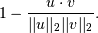# scipy.spatial.distance.cosine¶

scipy.spatial.distance.cosine(u, v)[source]

Computes the Cosine distance between 1-D arrays.

The Cosine distance between u and v, is defined aswhereis the dot product ofand.

Parameters: u : (N,) array_like Input array. v : (N,) array_like Input array. cosine : double The Cosine distance between vectors u and v.

#### Previous topic

scipy.spatial.distance.correlation

#### Next topic

scipy.spatial.distance.dice